# PSEB 5th Class Maths Solutions Chapter 4 Fractions Ex 4.2

Punjab State Board PSEB 5th Class Maths Book Solutions Chapter 4 Fractions Ex 4.2 Textbook Exercise Questions and Answers.

## PSEB Solutions for Class 5 Maths Chapter 4 Fractions Ex 4.2

Question 1.
Match the following: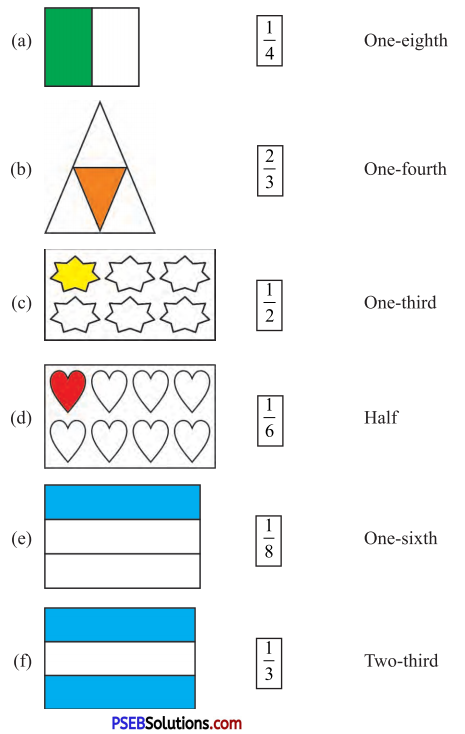Solution: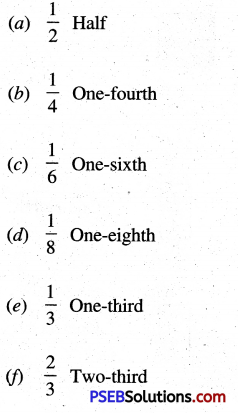Question 2.
Match the following: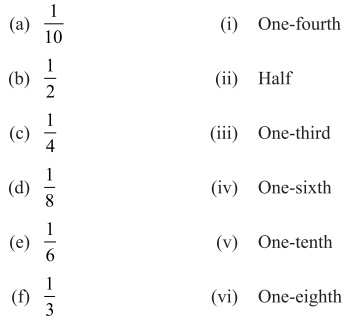Solution: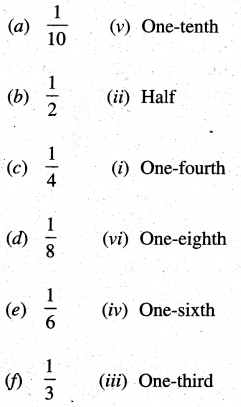Question 3.
Fill in the blanks:

(a) $$\frac{1}{3}$$ part of 9 guavas = _____ guavas
(b) $$\frac{1}{6}$$ part of 12 toffees = ___ toffees
(c) $$\frac{1}{6}$$ part of 18 ice-creams = ____ ice-creams
(d) $$\frac{1}{4}$$ part of 16 pencils = ___ pencils
(e) $$\frac{1}{10}$$ part of ₹ 20 = ₹ ___
(f) $$\frac{1}{10}$$ part of 100 pencils = ___ pencils
(g) $$\frac{1}{10}$$ part of 100 cm = ___ cm
(h) $$\frac{1}{8}$$ part of 32 laddoos = ___ laddoos.

Solution:

(a) $$\frac{1}{3}$$ × 9 = 3,
(b) $$\frac{1}{6}$$ × 12 = 2,
(c) $$\frac{1}{6}$$ × 18 = 3,
(d) $$\frac{1}{4}$$ × 16 = 4,
(e) $$\frac{1}{10}$$ × 20 = 2,
(f) $$\frac{1}{10}$$ × 100 = 10,
(g) $$\frac{1}{10}$$ × 100 = 10,
(h) $$\frac{1}{8}$$ × 32 = 2,

Question 4.
Neha’s uncle brought a chocolate which looks as following diagram: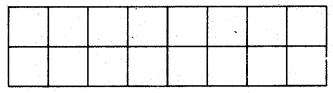(a) Neha gave half part of her chocolate to her sister Nidhi. How many pieces of chocolate will she give to Nidhi?
(b) Neha gave one-eighth part of this chocolate to her grand mother. How many pieces will she give to her?
(c) Neha gave one-fourth part of this chocolate to her mother. How many pieces will she give to her?
(d) After giving pieces to all, rest of pieces she ate. How many pieces will she get for herself?
Solution:
(a) Total number of pieces of chocolate = 16
Number of pieces given to Nidhi = $$\frac{1}{2}$$ × 16 = 8
(b) Number of pieces given to her grand
mother = $$\frac{1}{8}$$ × 16 = 2
(c) Number of pieces given to her mother = 16 × $$\frac{1}{4}$$ = 4
(d) Number of pieces she will get for herself = 16 – (8 + 2 + 4)
= 16 – 14 = 2 .Question 5.
Arjun studies in 5th class. He spends his day according to the following schedule.

• One fourth of a day in the school.
• One third of a day for sleeping.
• $$\frac{1}{12}$$th of a day for watching T.V.
• $$\frac{1}{12}$$th of a day for playing.
• $$\frac{1}{8}$$th of a day for school homework. 8
• $$\frac{1}{8}$$th of a day he spends with grand parents.

After the following :

(a) How much time Arjun spends in School ?
(b) For how many hours Arjun takes sleep ?
(c) For how many hours Arjun watches T.V. ?
(d) For how many hours Arjun plays ?
(e) For how many hours Aijun does his homework.
(f) How many hours Arjun spends with his grand parents ?
Note. Teacher will tell the students that there are 24 hours in a day.
Solution:
(a) Time spent by Aijun in the school = One fourth of a day.
= $$\frac{1}{4}$$ × 24 hours = 6 hours.
(b) Number of hours for which Aijun takes sleep = one third of a day
= $$\frac{1}{3}$$ × 24 hours = 8 hours.
(c) Number of hours for which Aijun watches T.V. = One-twelfth of a day
= $$\frac{1}{12}$$ × 24 hours = 2 hours.
(d) Number of hours for which Arjun , plays = One twelfth of a day
= $$\frac{1}{12}$$ × 24 hours = 2 hours.
(e) Number of hours for which Aijun does his homework = One eighth of a day
= $$\frac{1}{8}$$ × 24 hours = 3 hours.
(f) Number of hours Aijun spends with his grandparents = one eighth of a day
= $$\frac{1}{8}$$ × 24 hours = 3 hours.